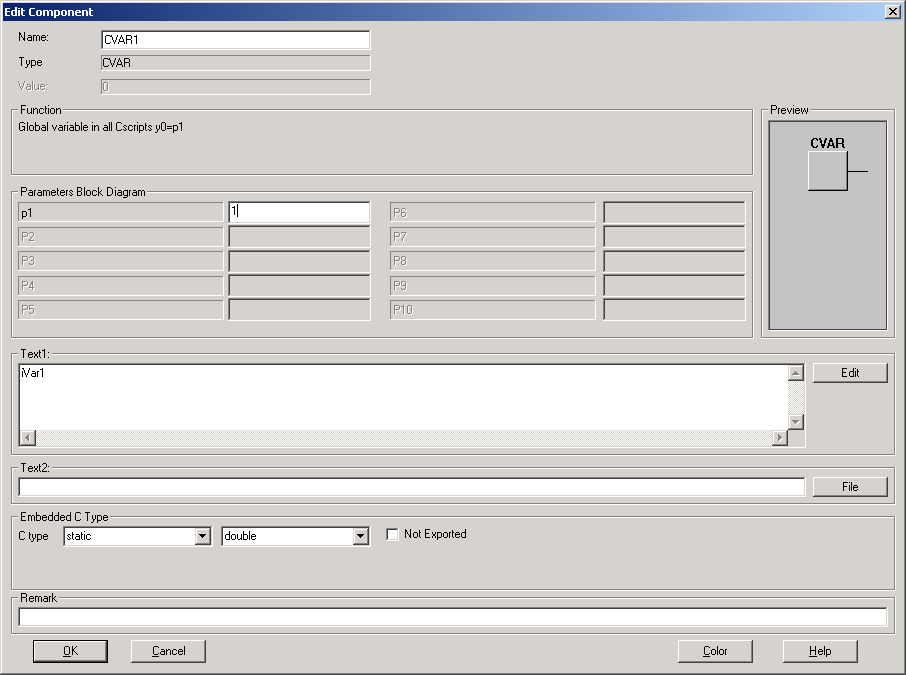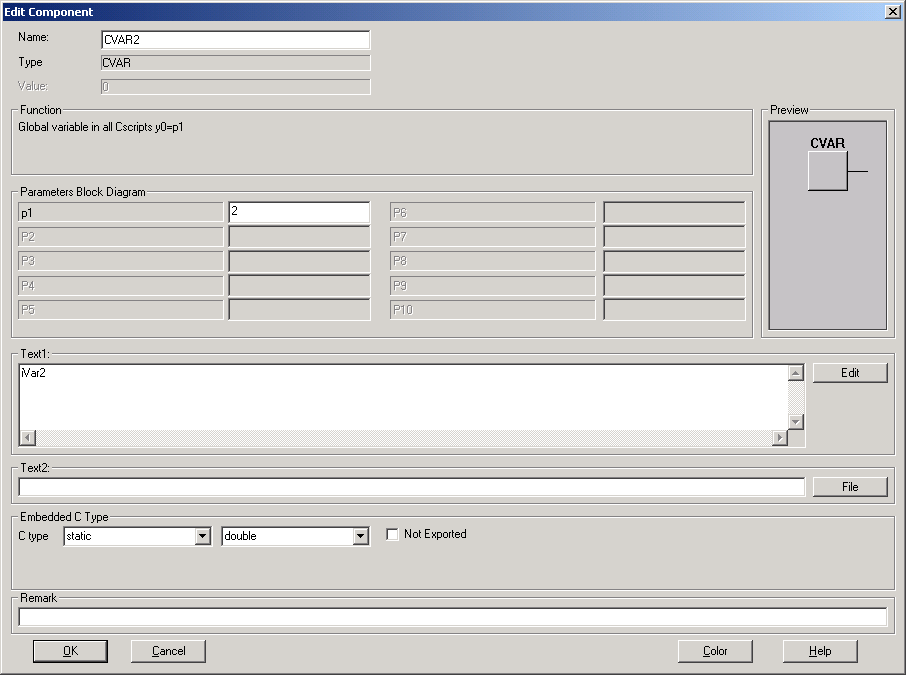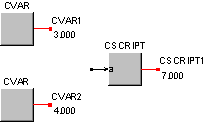• Introduction
• What is in this manual
• What is Caspoc
• User interface
• Introduction
• Starting
• Simulation
• Editing
• Viewing and printing
• Getting Started
• Basic editing
• Simulation in the time domain
• Basic User Interface Topics
• Editing
• Simulation
• Viewing
• Library
• Reports
• Project management
• Circuit and Block Diagram Components
• Introduction
• Cscript and user defined functions
• Component parameters
• Modeling Topics
• Introduction
• Power Electronics
• Semiconductors
• Electrical Machines
• Electrical drives
• Power Systems
• Mechanical Systems
• Thermal Systems
• Magnetic Circuits
• Green Energy
• Coupling to FEM
• Experimenter
• Analog hardware description language
• Embedded C code Export
• Coupling to Spice
• Small Signal Analysis
• Matlab coupling
• Tips and tricks
• Appendices

## Share variables among Cscripts

To exchange variables between Cscripts blocks or have more inputs and outputs, the CVAR block is used.

Using the CVAR block global variables are defined that are accessible in all Cscript blocks in your simulation.

Use this block to:

1. Send parameters to the Cscript blocks.
2. Get numerical values from the Cscript block.
3. Exchange numerical values between Cscript blocks
4. Provide more inputs & outputs for the Cscript blocks.

Per global variable one CVAR is used. Define the name of the variable at the edit field "text1" of the block.

In the ANSI-C language both variables "a" and "A" can exist and have different values. In other words, "a" and "A" are not equal. At p1 in the properties you can also define the initial value. The declared variable can be used in all Cscript blocks in your simulation.

In the example below two global variables are declared: iVar1 and iVar2, both of the type integer. Their default value is set to

  iVar1=1; iVar2=2; 

by defining the default value 1 at p1 for iVar1:by defining the default value 2 at p1 for iVar2:Both variables iVar1 and iVar2 are declared for all Cscripts in the simulation. In this simulation we will use the default value and replace it with a new value.

In the Cscript, the value of iVar1 and iVar2 is added and returned as the output of the Cscript block. The variables iVar1 and iVar2 get a new value being:

  iVar1=3; iVar2=4; 

and thereby replacing the previous values. These new values are set at the outputs of the CVAR blocks.

The Cscript is shown below.

  int y; main()    {    y=iVar1+iVar2;    iVar1=3;    iVar2=4;    return(y);    } 

After running the simulation the output of the Cscript block becomes equal to 7 except for the first time step, where the output will equal the summation of the default values 1+2.The outputs of the blocks CVAR are equal to 3 and 4, because of the assignment in line 5 and 6 in the Cscript.